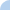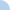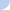Algebra 2: Simplifying Algebraic Fractions!

Remember that if you have a polynomial in either the numerator or denominator you need to FACTOR first, THEN CANCEL!!!!
Don't forget that there are two ways of factoring a polynomial -- common terms and also into two binomials.Quiz Log In